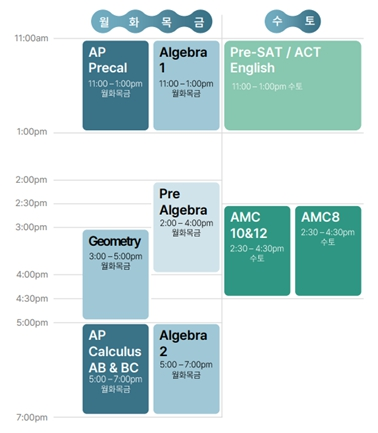알림

## 2023 여름특강

##### 국제학교 재학생들을 위한 양질의 수업을 제공합니다.

📅 개강 일정 📅

1차 : 6/12~7/15, 6/19~7/22

2차 : 7/17~8/19

#### INTENSIVE Math Practices

###### 에이샙은 국제학교 수학 선행학습과 한국 수학 문제지 풀이를 같이 병행하는 커리큘럼을 채택하고있습니다.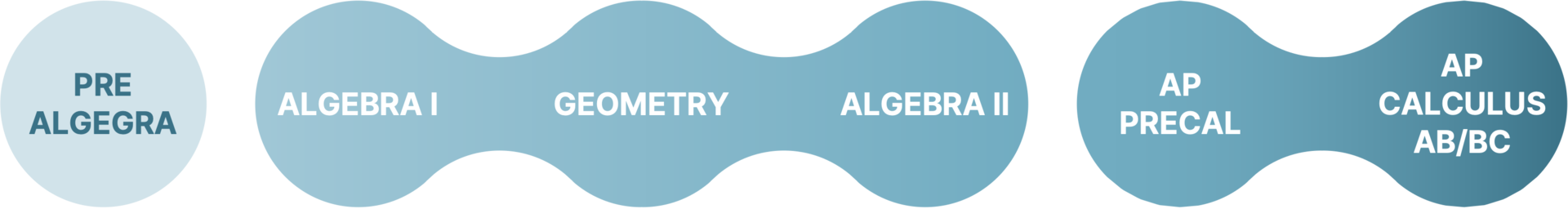#### 커리큘럼

Week 1 Week 2 Week 3 Week 4 Week 5
Day1
Variables, Expressions, and Integers
Day 1
Test + Review / Factors, Fractions, and Exponents
Day 1
Ratio, Proportion and Probability
Day 1
Real Numbers and Right Triangle
Day 1
Test + Review / Data Analysis and Probability
Day 2
Solving Equations
Day 2
Factors, Fractions, and Exponents
Day 2
Percents
Day 2
Real Numbers and Right Triangle
Day 2
Polynomials and Nonlinear Functions
Day 3
Multi-step Equations and Inequalities
Day 3
Rational Numbers and Equations
Day 3
Test + Review / Linear Functions
Day 3
Measurements, Area and Volume
Day 3
Angle Relationships and Transformations
Day 4
Multi-step Equations and Inequalities
Day 4
Rational Numbers and Equations
Day 4
Linear Functions
Day 4
Measurements, Area and Volume
Day 4
Final Exam + Review
Week 1 Week 2 Week 3 Week 4 Week 5
Day1
Algebra -
Fundamentals
Day 1
Systems of Equations: Intro
Day 1
Day 1
Completing
Formula
Day 1
Series: Arithmetic
Day 2
Linear Equation:
Introduction
Day 2
Systems of Equation: Graph
Day 2
Factorization
Day 2
Graph Transformation
Day 2
Series: Geometric
Day 3
Linear Function:
Graph
Day 3
Inequalities
Day 3
Day 3
Sequence: Arithmetic
Day 3
Exponents
Day 4
Review
+ Graph Cont'd
Day 4
Inequalities:
Graph / Abs Value
Day 4
Review
Day 4
Sequence: Geometric
Day 4
Review OR Seminar
Week 1 Week 2 Week 3 Week 4 Week 5
Day1
Geometry basics: Angle/Perimeter/Area
Day 1
Review of Week 1 + Practice problems
Day 1
Review of Week 2 + Practice problems
Day 1
3D Geometry
Day 1
Review of Week 4 + Practice problems
Day 2
Triangles
Day 2
Day 2
Circles
Day 2
Curved surfaces
Day 2
Total Review + Questions
Day 3
Trigonometry
Day 3
Polygon
Day 3
Circles practice problems
Day 3
Analytic Geometry
Day 3
Supplementary class
Day 4
Supplementary class
Day 4
Supplementary class
Day 4
Supplementary class
Day 4
Analytical Geometry
Day 4
Practice test + Review
Week 1 Week 2 Week 3 Week 4 Week 5
Day1
Quick Review of Algebra I
Day 1
Introduction to Polynomials: Essentials
Day 1
Rational Functions
Day 1
Introduction to Trigonometry: What is Trigonometry
Day 1
Trigonometry Review
Day 2
Introduction to Algebra II: Linear Functions
Day 2
Factorization / Division
Day 2
Exponential Functions
Day 2
Understanding Trigs Through Unit Circle
Day 2
Probability
Day 3
Day 3
Graphing Polynomials (Graph Transformation)
Day 3
Exponential Functions + Introduction to Logarithm
Day 3
Trigonometry Derivation
Day 3
Probability Seminar
Day 4
Graph Transformation
Day 4
Rational Functions
Day 4
Logarithmic Function
Day 4
Trigonometry Identities
Day 4
Review
Week 1 Week 2 Week 3 Week 4 Week 5
Day1
Polynomial Function / Graphs
Day 1
Logarithmic Functions: Law of Logarithm
Day 1
Trigonometry Formulas
Day 1
Vector
Day 1
Complex number (De Moivre's)
Day 2
Dividing Polynomial (Real Zero)
Day 2
Modelling Logarithmic Function
Day 2
Inverse Trigonometry
Day 2
Conic section
Day 2
Limit
Day 3
Complex Numbers & Zeroes
Day 3
Trigonometry: Unit Circle
Day 3
Sequence
Day 3
Matrix: Systems of Equations of Multiple Variables
Day 3
Review
Day 4
Exponential Function / Graph
Day 4
Trigonometry Identities
Day 4
Polar Function Graph
Day 4
Inverse Matrix and Determinant
Day 4
Final Exam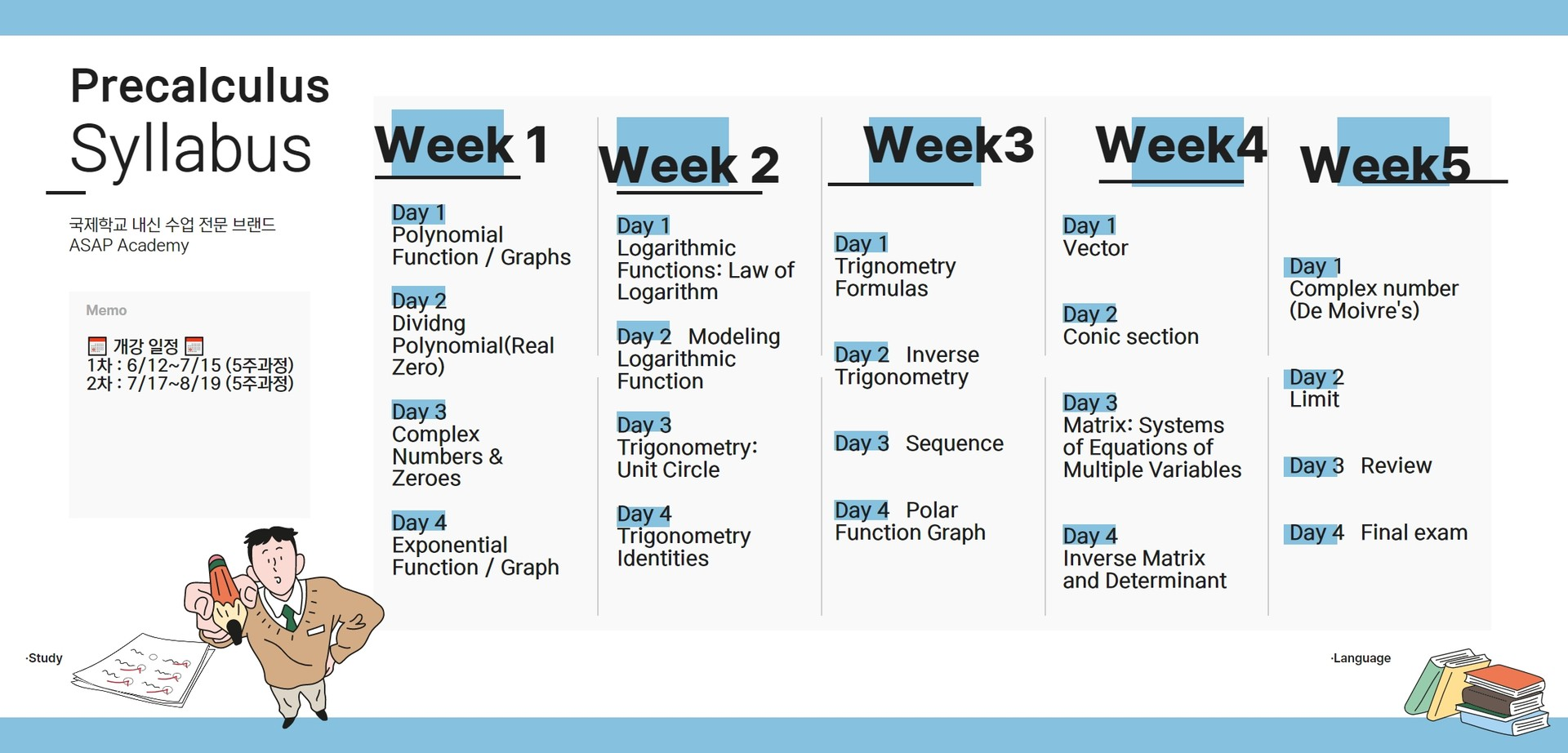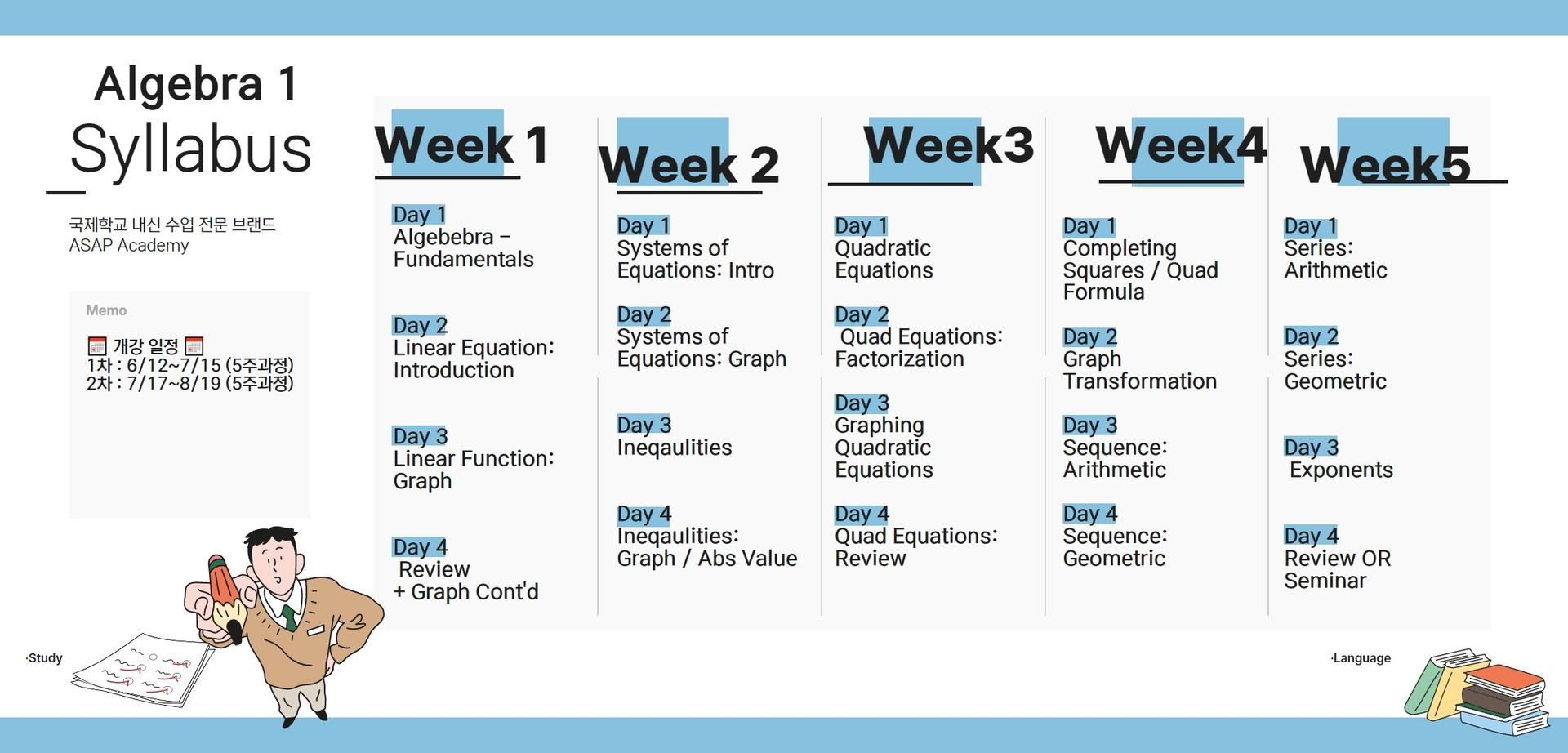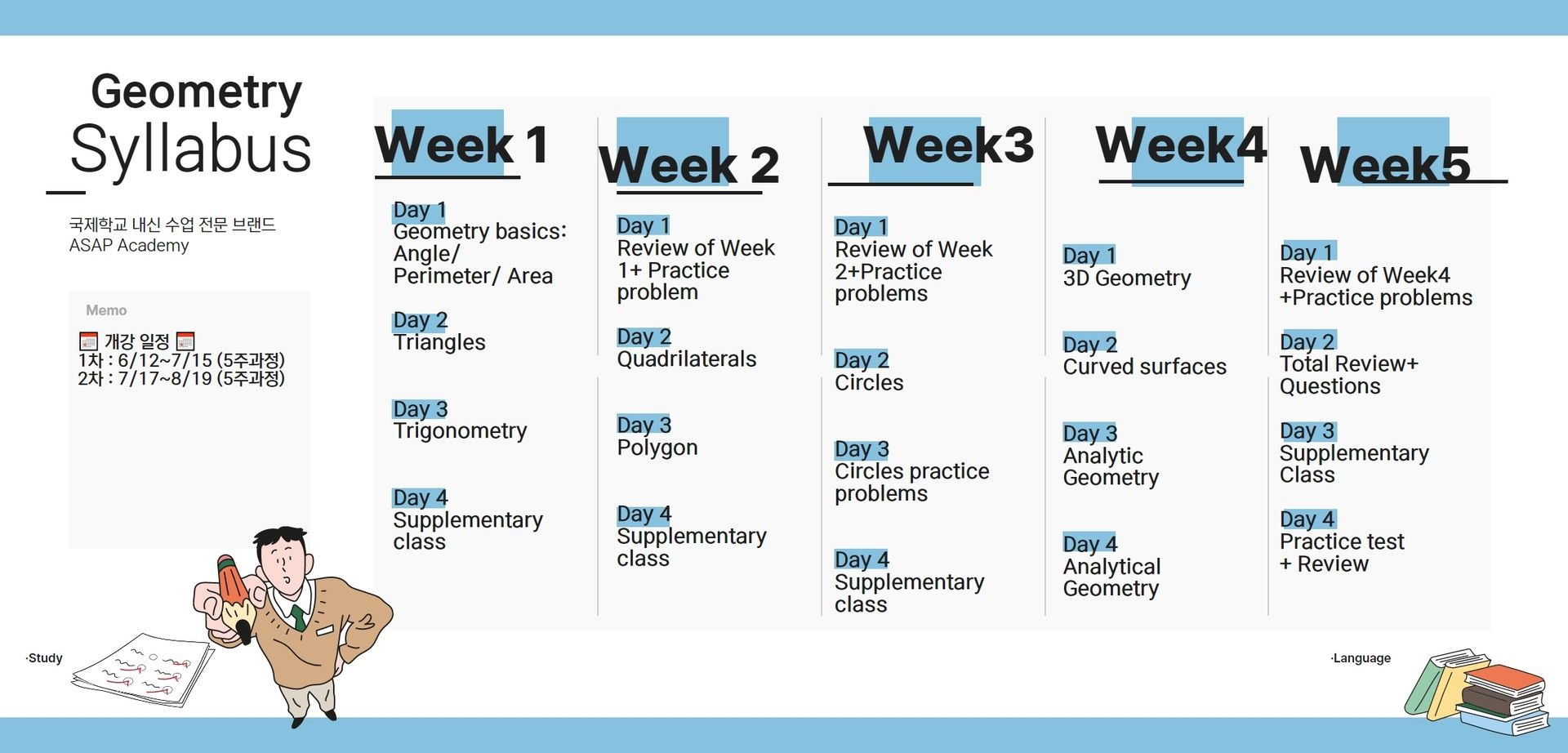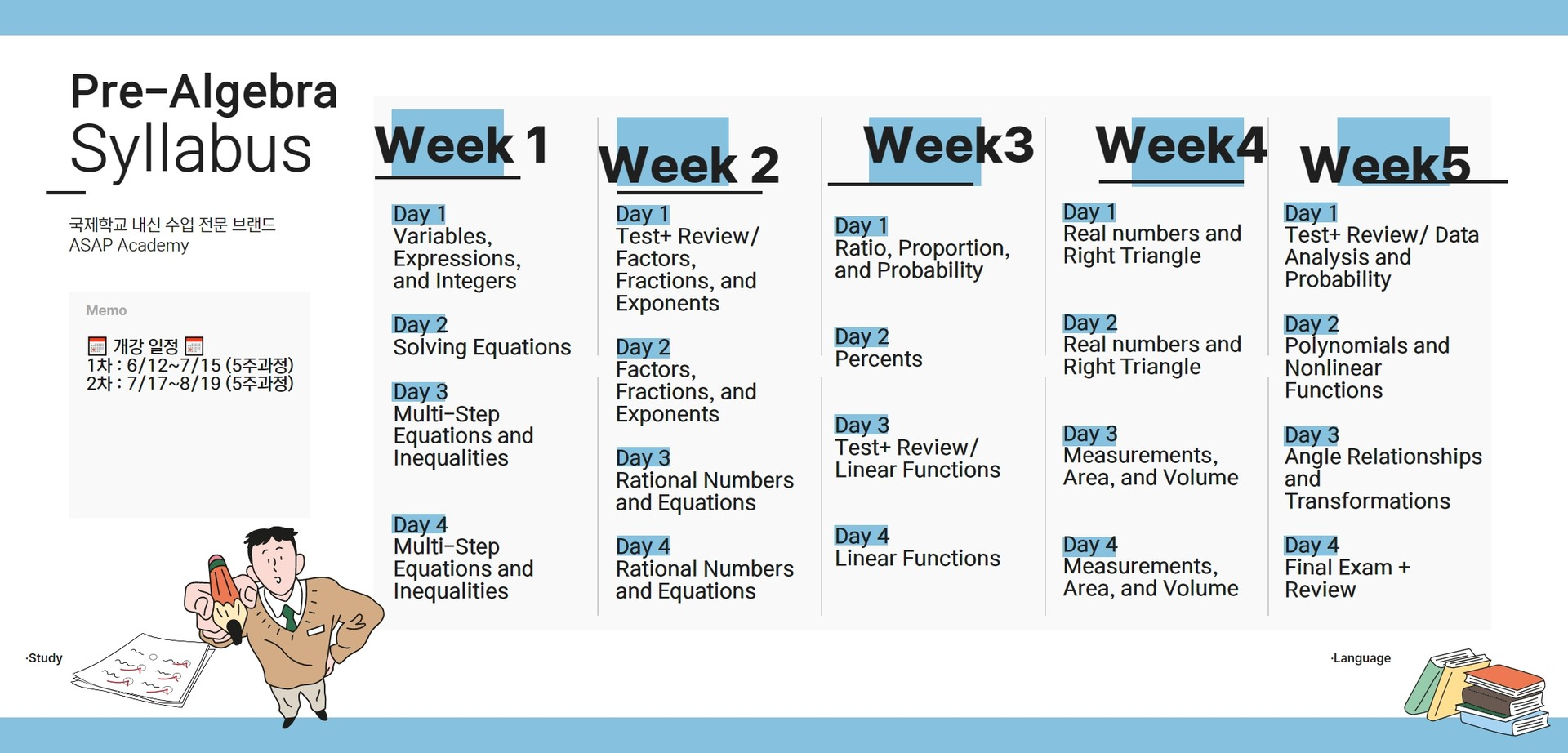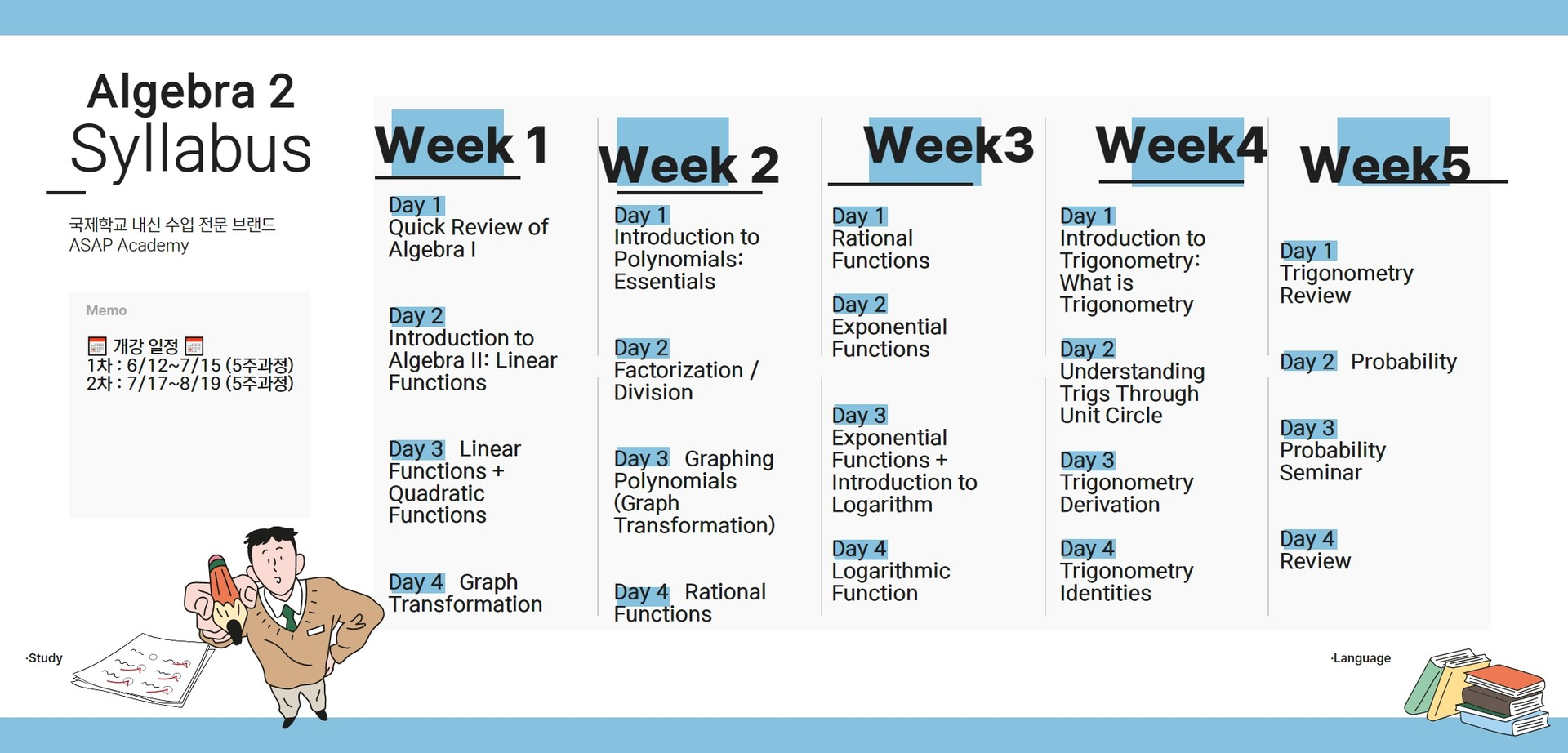**특강 등록 안내

정원은 8명 입니다.

환불은 서울시 교육청 학원환불규정을 따릅니다.

#### Pre SAT/ACT English

###### 작문훈련과 독해실력을 기본부터 탄탄하게 쌓아올리는 수업을 진행합니다.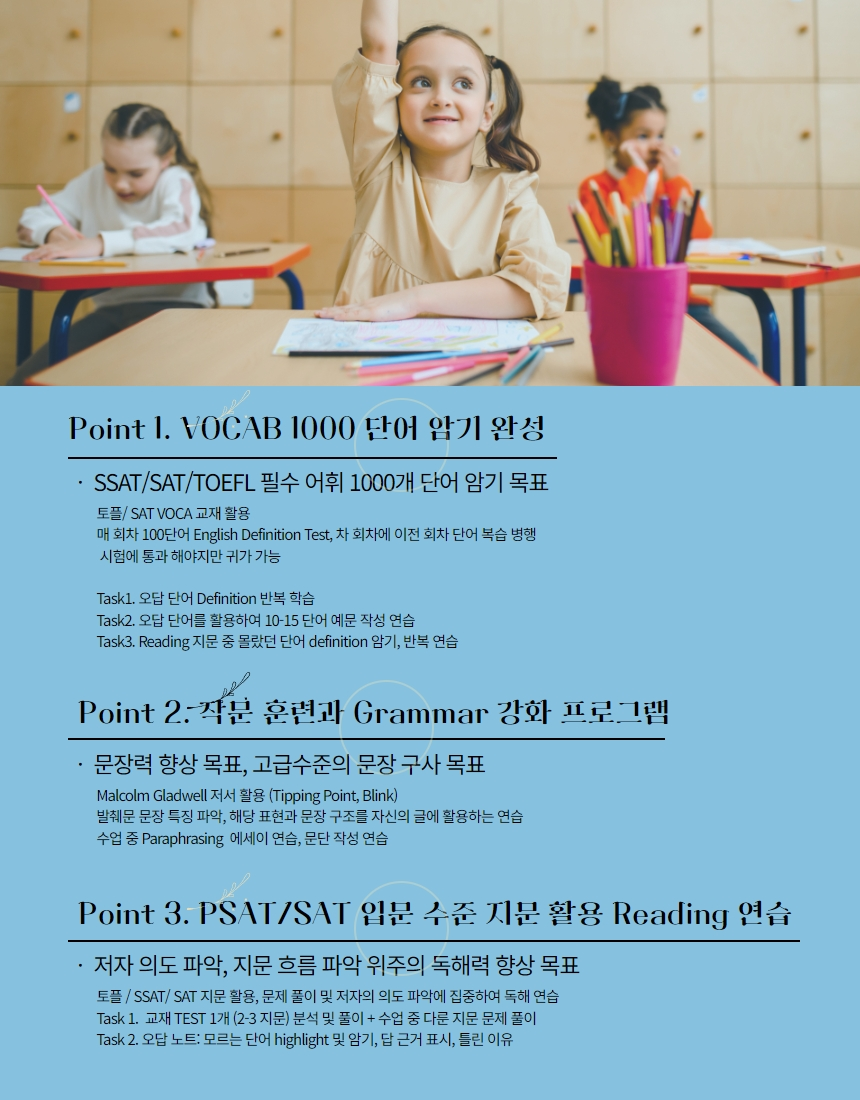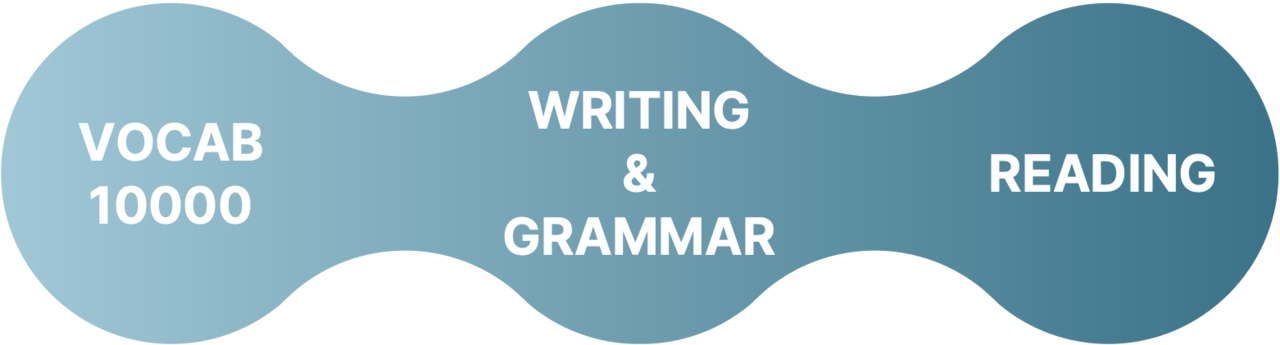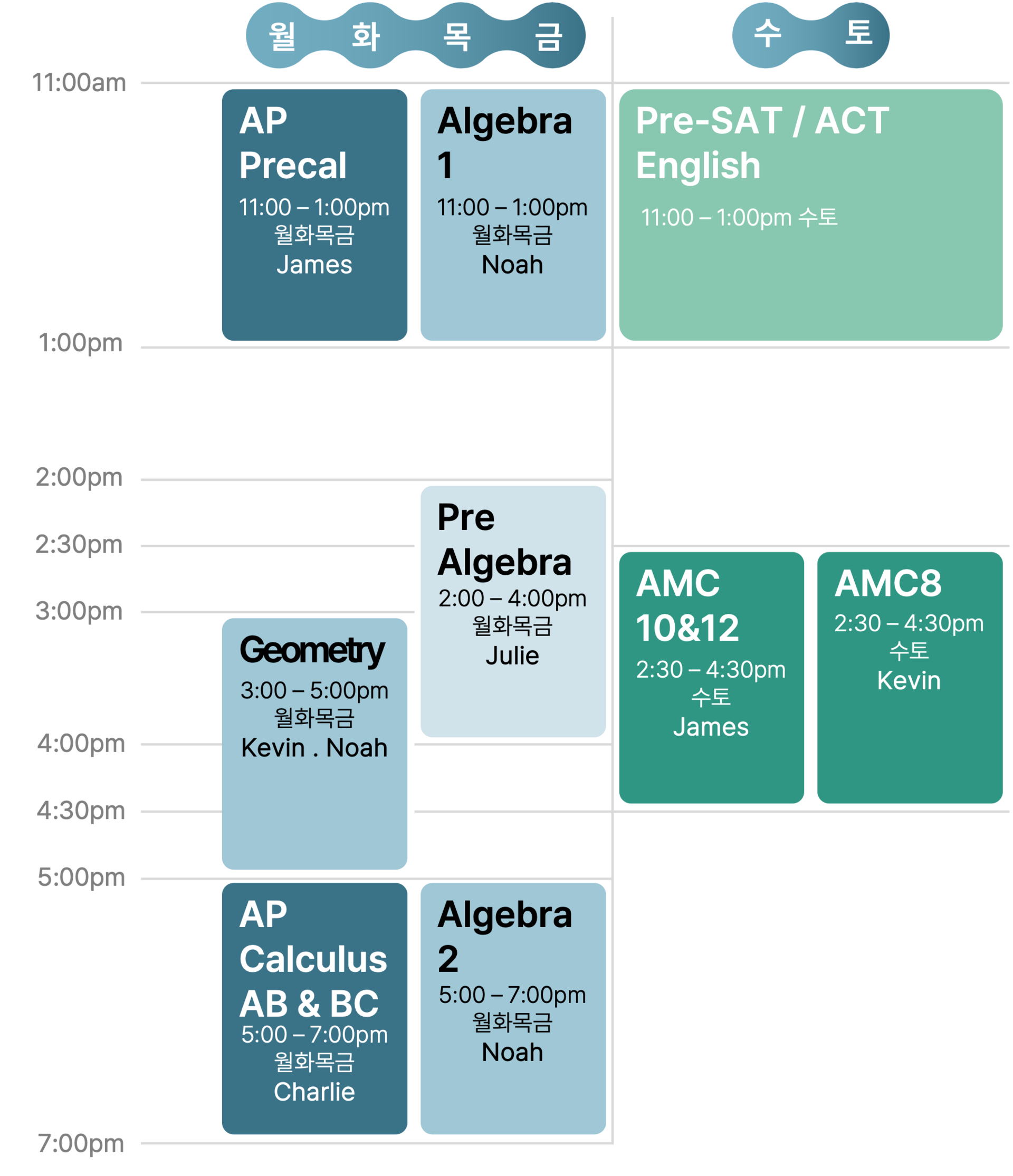📅 개강 일정 📅

1차 : 6/12~7/15, 6/19~7/22, 6/26~7/29

2차 : 7/17~8/19